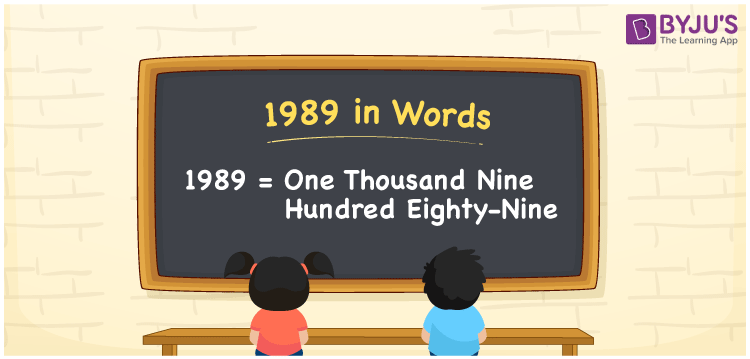# 1989 in Words

1989 in words is written as One thousand nine hundred eighty-nine. If you spend a bill of Rs. 1989 for mobile postpaid service, you can say, “I spent One thousand nine hundred eighty-nine as my mobile postpaid bill”. Also, we use the word form of the cardinal number 1989 in many conversations in our daily existence. Thus, the number name of 1989 is known as One thousand nine hundred eighty-nine.

 1989 in words One thousand nine hundred eighty-nine One thousand nine hundred eighty-nine in Numbers 1989

## 1989 in English Words

Using the English alphabet, we can express numbers in words. So, we can spell 1989 in English as “One thousand nine hundred eighty-nine”.## How to Write 1989 in Words?

We can easily write the number 1989 in words with the help of a place value chart. The below table shows the place values for all the digits of 1989.

 Thousands Hundreds Tens Ones 1 9 8 9

Here, ones = 9, tens = 8, hundreds = 9, and thousands = 1.

Thus, we can write the expanded form as:

1 × Thousand + 9 × Hundred + 8 × Ten + 9 × One

= 1 × 1000 + 9 × 100 + 8 × 10 + 9 × 1

= 1000 + 900 + 80 + 9

= One thousand + Nine hundred + Eighty + Nine

= One thousand nine hundred eighty-nine

Therefore, 1989 in words = One thousand nine hundred eighty-nine.

As we know, 1989 is a natural number that precedes 1990 and succeeds 1988.

1989 in words – One thousand nine hundred eighty-nine

Is 1989 an even number? – No

Is 1989 an odd number? – Yes

Is 1989 a prime number? – No

Is 1989 a composite number? – Yes

Is 1989 a perfect square number? – No

Is 1989 a perfect cube number? – No

## Frequently Asked Questions on 1989 in Words

### How do you write 1989 in words?

The number 1989 can be written in words as One thousand nine hundred eighty-nine.

### How do you spell 1989?

You can spell the number 1989 using English words as “One thousand nine hundred eighty-nine”.

### Write Rs. 1989 amount in words.

We can write an amount of Rs. 1989 in words as “One thousand nine hundred eighty-nine rupees”.